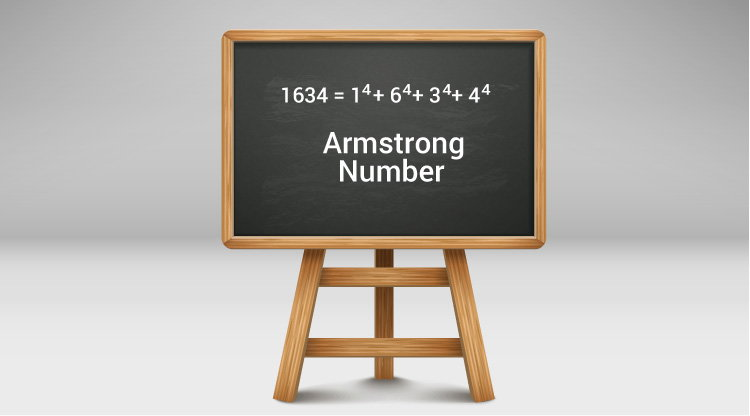C Program to Display Armstrong Number Between Two Intervals

Example to find all Armstrong numbers between two integers (entered by the user) using loops and if...else statement.To understand this example, you should have the knowledge of following C programming topics:

A positive integer is called an Armstrong number of order n if

abcd... = an + bn + cn + dn + ...

In case of an Armstrong number of 3 digits, the sum of cubes of each digits is equal to the number itself. For example:

153 = 1*1*1 + 5*5*5 + 3*3*3  // 153 is an Armstrong number.

This program is built on the concept of how to check whether an integer is an Armstrong number or not.

Example: Armstrong Numbers Between Two Integers

#include <stdio.h>
#include <math.h>

int main()
{
int low, high, i, temp1, temp2, remainder, n = 0, result = 0;

printf("Enter two numbers(intervals): ");
scanf("%d %d", &low, &high);
printf("Armstrong numbers between %d an %d are: ", low, high);

for(i = low + 1; i < high; ++i)
{
temp2 = i;
temp1 = i;

// number of digits calculation
while (temp1 != 0)
{
temp1 /= 10;
++n;
}

// result contains sum of nth power of its digits
while (temp2 != 0)
{
remainder = temp2 % 10;
result += pow(remainder, n);
temp2 /= 10;
}

// checks if number i is equal to the sum of nth power of its digits
if (result == i) {
printf("%d ", i);
}

// resetting the values to check Armstrong number for next iteration
n = 0;
result = 0;

}
return 0;
}

Output

Enter two numbers(intervals): 999
99999
Armstrong numbers between 999 an 99999 are: 1634 8208 9474 54748 92727 93084